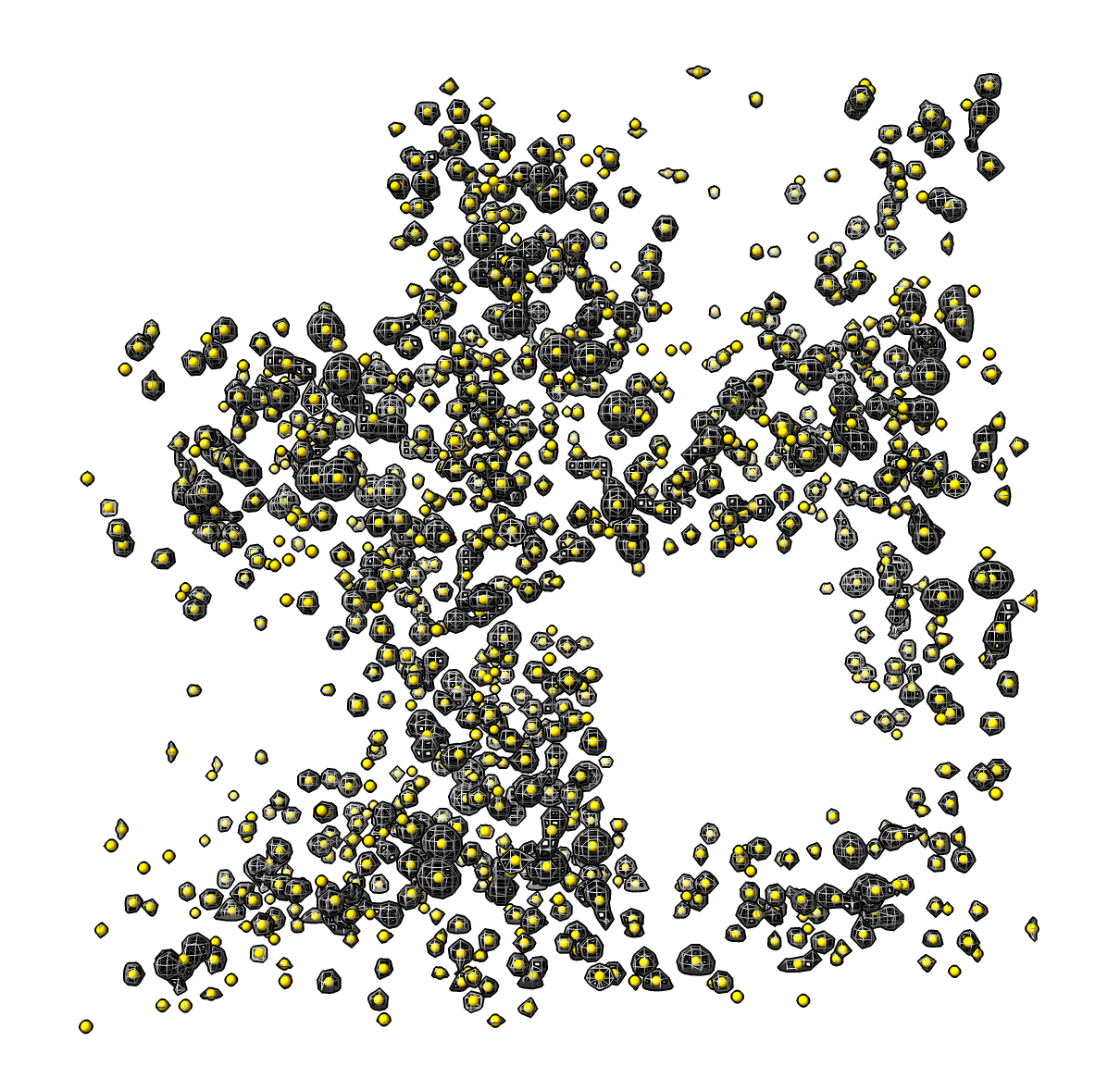# Mark Connected Pieces of a Surface

Here is some ChimeraX Python code that puts a spherical marker at the center of each connected piece of a surface. Brandon Scott asked about this for tracking blobs in 3D light microscopy. I pieced to together this code by looking at two existing ChimeraX features: the mouse mode for placing a marker at the center of a connected surface piece, and the surface dust command that hides all small connected surface pieces.

This capability has been added to the ChimeraX distribution as the mark connected command.

The Python code mark_blobs.py when opened in ChimeraX defines the markblobs command. Here is an example use marking each blob of the X-ray density for PDB crystal structure 1a0m

``````open ~/Downloads/mark_blobs.py
open 1a0m from eds
markblobs #1 radius 0.2 color gold
``````Here is the bit that computes the centers

```def surface_blob_centers(surface):
'''
Return an array of center positions for each connected set of
displayed triangles in the surface.  Centers are in surface coordinates.
A center is computed as average vertex position weighted by vertex area
where vertex area is 1/3 the area of the adjoining displayed triangles.
'''

# Get list of (vertex indices, triangle indices) for each connected piece
from chimerax.surface import connected_pieces, vertex_areas
blob_list = connected_pieces(triangles)

# Compute area-weighted center for each blob
vertices = surface.vertices
varea = vertex_areas(vertices, triangles)
from numpy import empty, float32
centers = empty((len(blob_list), 3), float32)
for i, (vi,ti) in enumerate(blob_list):
blob_varea = varea[vi]
blob_area = blob_varea.sum()
centers[i] = blob_varea.dot(vertices[vi])/blob_area

return centers
```

And here is some Python to define a command “markblobs” that runs this.

```def markblobs(session, surface, radius = 0.5, color = (255,255,0,255)):
centers = surface_blob_centers(surface)
scene_centers = surface.scene_position * centers
from chimerax.markers import MarkerSet
marker_set = MarkerSet(session, name = surface.name)
markers = [marker_set.create_marker(center, color, radius) for center in scene_centers]
session.logger.status('Found %d connected surface pieces' % len(markers), log = True)
return markers

def register_command(logger):
from chimerax.core.commands import CmdDesc, register, SurfaceArg, FloatArg, Color8Arg
desc = CmdDesc(
required = [('surface', SurfaceArg)],